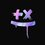# Unsolved Questions - 3

Where's the remaining $1,000? This is a problem which is really hard to understand how it ends. This is how it goes; You have 2 friends, John and Anna. You borrow$50,000 from both, which means you get a sum of $100,000. You buy a laptop which costs$97,000, so then you have $3,000 remaining. You give$1,000 to John, and another $1,000 to Anna, and you keep the remaining$1,000 for yourself. Now, if calculate this mathematically, you have actually only taken $49,000 from John, and$49,000 from Anna. Here comes the problem. $49,000 +$49,000 = $98,000... Right? But then you had$1,000 with you. So if we add those two like this; $98,000 +$1,000, you get $99,000. WHAT? Where's the other thousand? How did$100,000 become $99,000 at the end?? My father told me, that to find the answer to solve this problem, you need to use "Reverse Phsycology". But how? If you had found a way to unknot this knoted problem, please leave your answer below. If you haven't, just leave your thoughts about this below :)Note by Hasna Hassen 1 year, 2 months ago This discussion board is a place to discuss our Daily Challenges and the math and science related to those challenges. Explanations are more than just a solution — they should explain the steps and thinking strategies that you used to obtain the solution. Comments should further the discussion of math and science. When posting on Brilliant: • Use the emojis to react to an explanation, whether you're congratulating a job well done , or just really confused . • Ask specific questions about the challenge or the steps in somebody's explanation. Well-posed questions can add a lot to the discussion, but posting "I don't understand!" doesn't help anyone. • Try to contribute something new to the discussion, whether it is an extension, generalization or other idea related to the challenge. • Stay on topic — we're all here to learn more about math and science, not to hear about your favorite get-rich-quick scheme or current world events. MarkdownAppears as *italics* or _italics_ italics **bold** or __bold__ bold - bulleted- list • bulleted • list 1. numbered2. list 1. numbered 2. list Note: you must add a full line of space before and after lists for them to show up correctly paragraph 1paragraph 2 paragraph 1 paragraph 2 [example link](https://brilliant.org)example link > This is a quote This is a quote  # I indented these lines # 4 spaces, and now they show # up as a code block. print "hello world" # I indented these lines # 4 spaces, and now they show # up as a code block. print "hello world" MathAppears as Remember to wrap math in $$ ... $$ or $ ... $ to ensure proper formatting. 2 \times 3 $2 \times 3$ 2^{34} $2^{34}$ a_{i-1} $a_{i-1}$ \frac{2}{3} $\frac{2}{3}$ \sqrt{2} $\sqrt{2}$ \sum_{i=1}^3 $\sum_{i=1}^3$ \sin \theta $\sin \theta$ \boxed{123} $\boxed{123}$ ## Comments Sort by: Top Newest In the end, John has given 49,000 and Anna has given 49,000, for a total of 98,000. The laptop store has 97,000 of that , and you have 1000, for a total of 98,000. It all adds up - 1 year, 2 months ago Log in to reply Thanks alot :D - 1 year, 2 months ago Log in to reply @Steven Chase had a great explanation. Here's another way of thinking about it: At the beginning - You:$0 | John: $50,000 | Anna:$50,000 | Laptop Store: $0 | Total =$100,000

After borrowing money - You: $100,000 | John:$0 | Anna: $0 | Laptop Store:$0 | Total = $100,000 After buying the laptop - You:$3000 | John: $0 | Anna:$0 | Laptop Store: $97,000 | Total =$100,000

Finally, after paying John and Anna - You: $1000 | John:$1000 | Anna: $1000 | Laptop Store:$97,000 | Total = \$100,000

Hope this helps!

- 1 year, 2 months ago

Wow :0 I had no idea that this simple explanation would be the answer!

- 1 year, 2 months ago